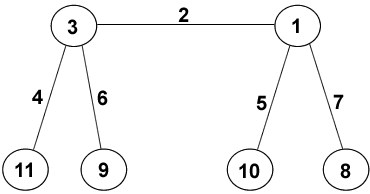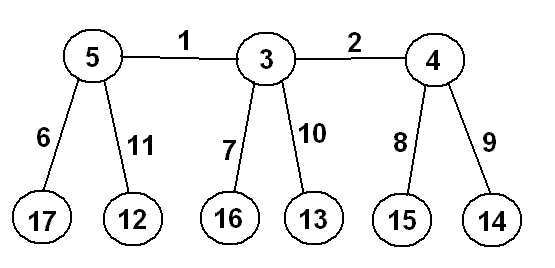#### You may also like### Instant Insanity

Given the nets of 4 cubes with the faces coloured in 4 colours, build a tower so that on each vertical wall no colour is repeated, that is all 4 colours appear.### Tree Graphs

A connected graph is a graph in which we can get from any vertex to any other by travelling along the edges. A tree is a connected graph with no closed circuits (or loops. Prove that every tree has exactly one more vertex than it has edges.### Plum Tree

Label this plum tree graph to make it totally magic!

# Magic Caterpillars

##### Age 14 to 18 Challenge Level:

Peter sent us this solution, using the starting point we gave:

There are $6$ vertices and $5$ edges. Let's suppose the magic constant is $S$ and this is the same at each vertex. The total of all the numbers $1 + 2 + ... + 11 = 66$ but the numbers on the edges, $a,b,c,d,e$ are counted twice. So, adding the magic sum at all six vertices:

$$(a+b+c+d+e)+66=6S$$

So $a+b+c+d+e$ is a multiple of $6$ and it is at least $1+2+3+4+5=15$ so it must be $18$ or more. Hence $6S\geq84$ and $S\geq14$.

We're going to call the horizontal edge $e$, the front two edges $a$ and $b$, and the back two legs $c$ and $d$. Also, we see that the numbers $11$, $10$ and $9$ must all be in feet, as they're too big to be anywhere else.

Let's try $S=14$ first. We may as well take $a=3$, as the legs are all interchangeable at this stage.

Now if $b=4$, then we have $c=5$ (as the back legs are interchangeable). Also, we must have $d$ and the back foot as $6$ and $8$ (in some order), and we soon see that this isn't possible (as we'd also need $e$ plus the top left hip to add up to $7$). If $b$ isn't $4$, then we can assume that $c=4$. But similarly we find that we can't have $b=9$ or $b=8$ or $b=7$. So $S$ isn't $14$.

Let's try $S=16$. But then we need four pairs of numbers that sum to $16$ (one for each leg). We try: $(11,5)$, $(10,6)$, $(9,7)$, $(8,?)$ and we can't repeat the $8$ so that isn't possible.

So let's try $S=15$. This time, we may as well take $a=4$. There are only $4$ pairs that add up to $15$: $(11,4)$, $(10,5)$, $(9,6)$ and $(8,7)$. Call the number in the top left hip $f$.

If $b=5$, then we have $c=6$ and $e+f=6$, which isn't possible (as we don't have the right numbers left).

So we can take $c=5$. If $b=8$, have $e+f=3$ and then we'll need $f+g=4$, and this isn't possible. If $b=7$, have $e+f=4$ and we also have $f+g=4$ and this isn't possible either. Also we can't have $d=8$, as this is too big. So the only possibility is the one shown below (and this does indeed work).

(When I say the only possibility, of course the front legs could be switched, or the back legs, or the front and back swapped, but it's really the same caterpillar.)West Lakes Academy year 10 MadMathsClub tried to prove our claim that there was no magic 6-legged caterpillar. In fact, we were wrong! They found a magic 6-legged creature as shown below:whose vertex-sums are all 23. Good work!